# Division: 4-Digit Dividend, 1-Digit Divisor

Times up!
Times up! You may finish answering the current question and then proceed to your score!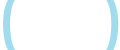Sorry, you have used all of your available hints for this lesson.
Practice Limit Reached

You've reached your daily practice limit of 25 questions.

Are you over 18 years old?
Award InformationAwarded toQuestion HintChoose Family Member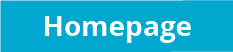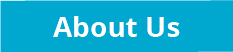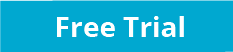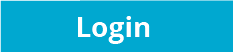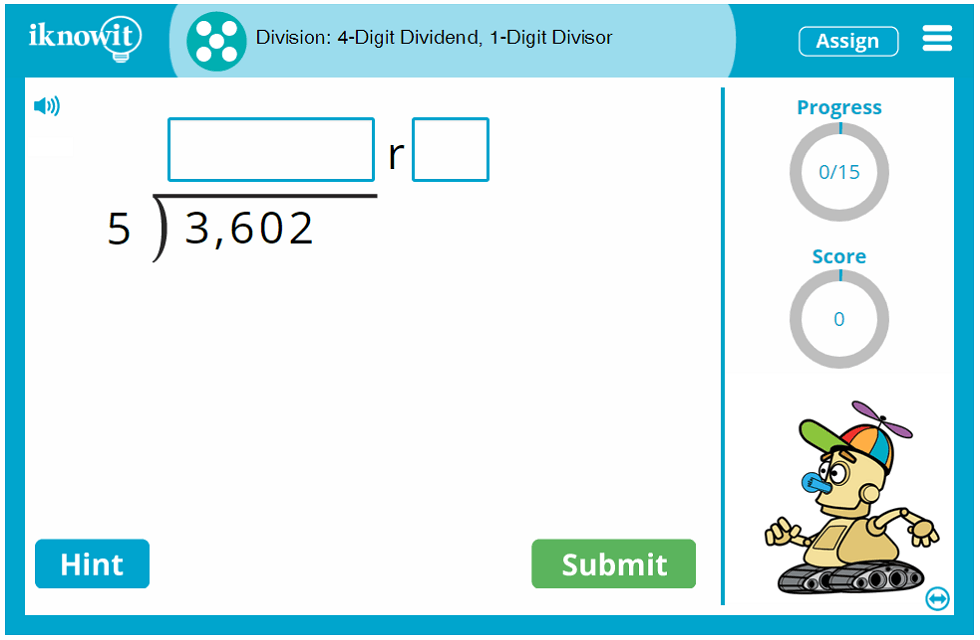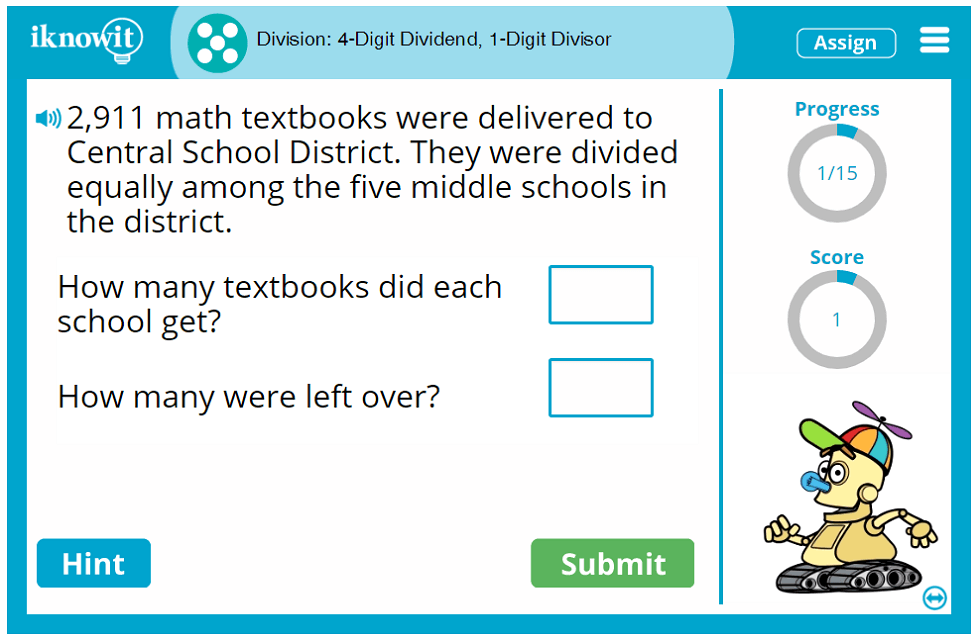## Interactive Math Activity - Dividing 4-Digit Dividends by 1-Digit Divisors

Get ready to practice division skills with your students! This fifth grade interactive math lesson will turn your students into division experts in no time! Students will solve for the quotient and remainder in a variety of math problems that will challenge their division skills. In addition to traditional-format division problems, students will be asked to solve division word problems as well.

Here are some examples of math problems students may be asked in this division lesson: "3,602/5 =" "4,983/7 =" and "Minty Fresh Toothpaste Company distributed 2,874 samples of toothpaste evenly to five local dentist offices. How many samples did each office get? How many samples were left over?"

If students need a little extra help solving the division problems in this online math lesson, they can click the hint button, which will show them the first step in solving the problem: dividing the hundreds. If students answer a question incorrectly, they will be shown a detailed explanation page that takes them through all the steps to solving the division problem the right way: dividing the hundreds, dividing the tens, and dividing the ones.

Other features in our math lessons, including this fifth grade division lesson, that help students make the most out of their math practice time include: a progress-tracker that shows students how many questions they have answered so far; a score-tracker that shows students how many questions they have answered correctly; a read-aloud button that children can use to hear the question read to them in a clear voice, and more!

We hope you will try out this interactive division activity with your class, as well as our extensive collection of other math topics. We're confident that once you try our math practice program, you will notice the difference in your students' confidence and proficiency in their math skills!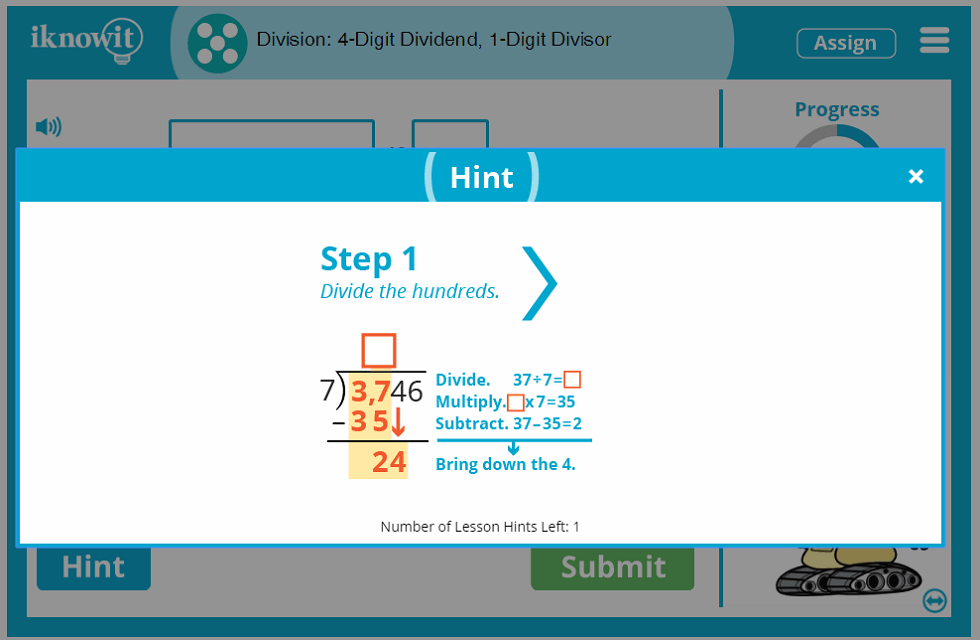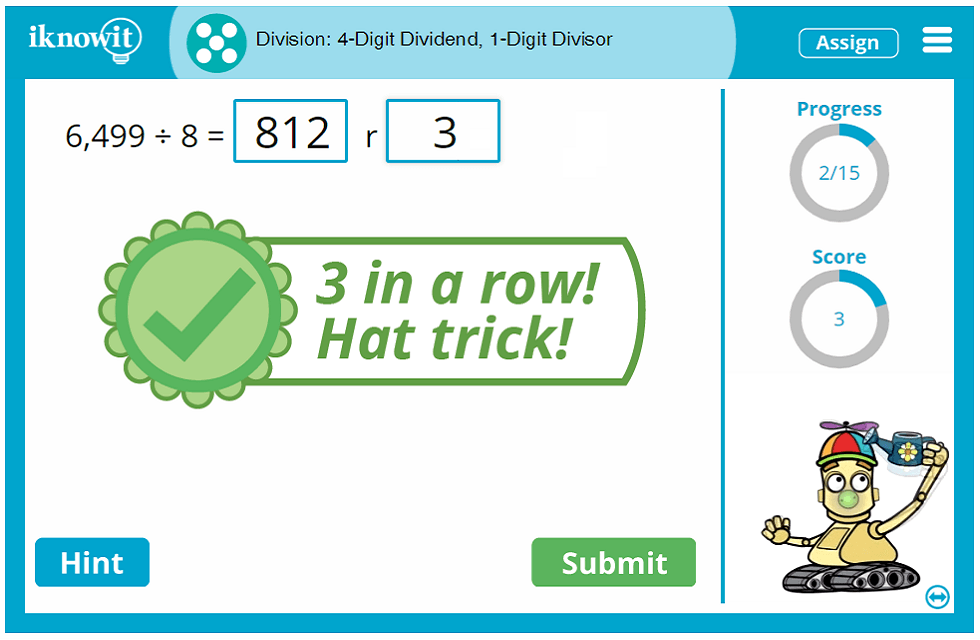## Free Trial and Membership Options

Take advantage of our free sixty-day trial offer, and try out this interactive division lesson with your class today! Your students will be able to check out any of our math practice activities in the free trial mode, but please know they will be capped at a total of twenty-five questions per day across all math lessons. For full and complete access to "I Know It," you will need to become a paying member of the website.

Grade levels in the student mode of "I Know It" are labeled with letters of the alphabet, so it's easy for you to assign math lessons based on skill level.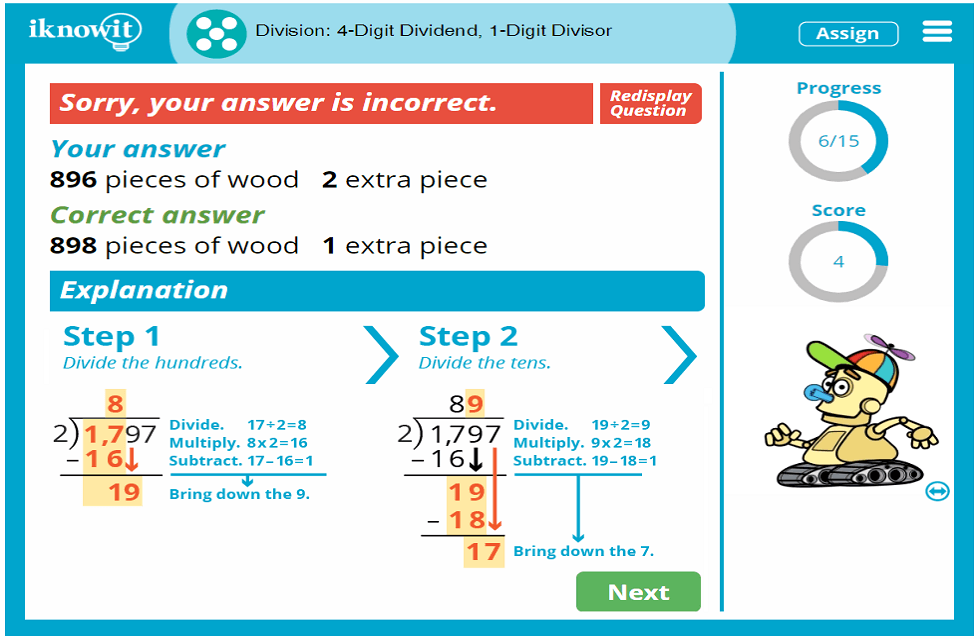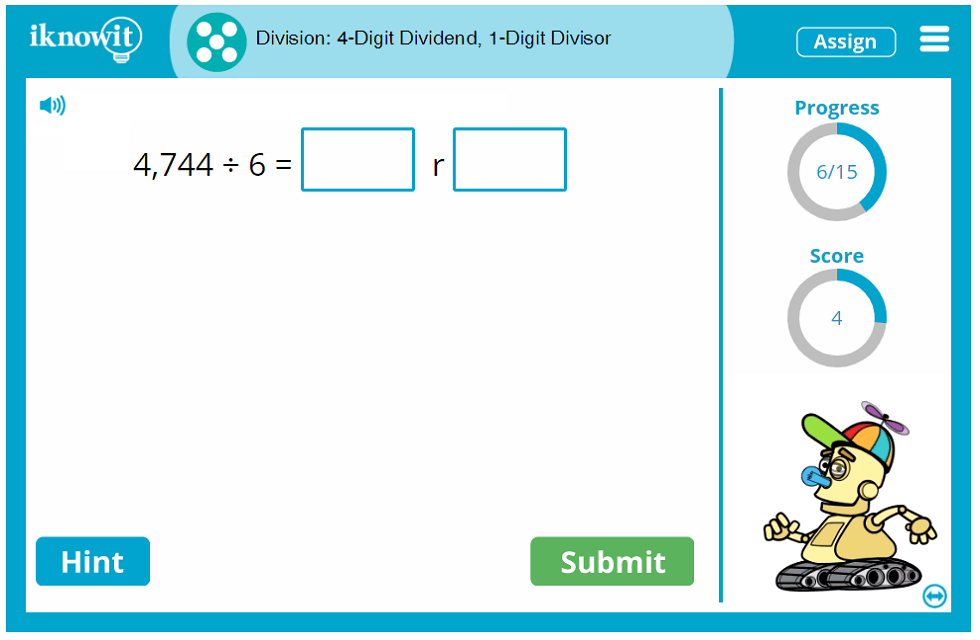## Level

This online math lesson is categorized as Level E. It may be ideal for your fifth grade class.

## Common Core Standard

5.NBT.B.6
Number and Operations in Base Ten

Students will perform operations with multi-digit whole numbers and with decimals to hundredths. Students will find whole-number quotients of whole numbers with up to four-digit dividends and two-digit divisors, using strategies based on place value, the properties of operations, and/or the relationship between multiplication and division.

## You might also be interested in...

Division: 4-Digit Dividend, 2-Digit Divisor (Level E)
In this fifth grade-level math lesson, students will practice division with four-digit dividends and 2-digit divisors. Students will solve for the quotient and remainder. Word problems are included in this interactive division lesson.

Dividing Whole Numbers by 10, 100, or 1,000 (Level E)
Students will practice dividing whole numbers by 10, 100, or 1,000 in this interactive math practice activity at a fifth grade level. Math problems include multiple choice, drag-and-drop, and fill-in-the-blank.